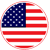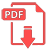# Home | Mathematics | Trigonometry

## Trigonometry Formulas

Trigonometry (from Greek trigōnon, "triangle" and metron, "measure") is a branch of mathematics that studies relationships between side lengths and angles of triangles. The field emerged in the Hellenistic world during the 3rd century BC from applications of geometry to astronomical studies. The Greeks focused on the calculation of chords, while mathematicians in India created the earliest-known tables of values for trigonometric ratios (also called trigonometric functions) such as sine.

Throughout history, trigonometry has been applied in areas such as geodesy, surveying, celestial mechanics, and navigation.English